Скачать презентацию Chapter 4 Demand Understanding Demand What

e28db30b25b0d2c06f6b4d3f44b1a870.ppt

• Количество слайдов: 23Chapter 4 Demand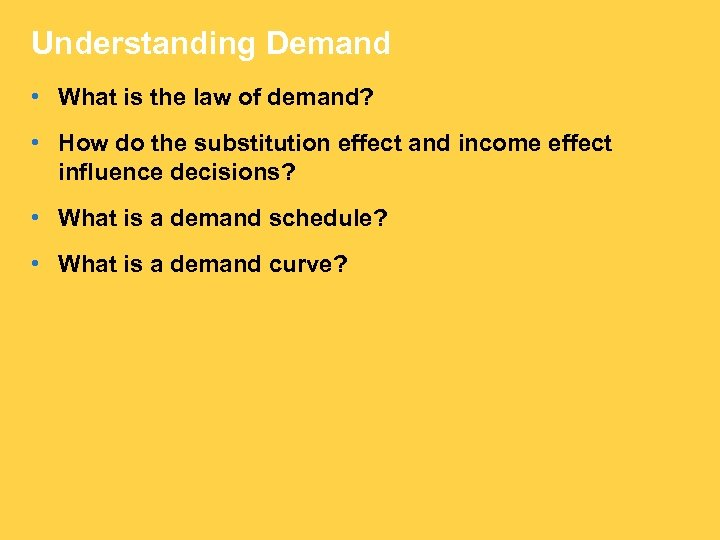Understanding Demand • What is the law of demand? • How do the substitution effect and income effect influence decisions? • What is a demand schedule? • What is a demand curve?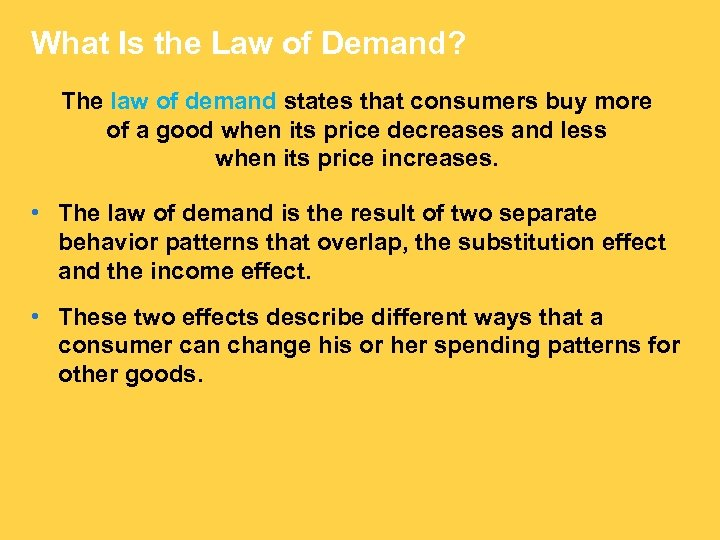What Is the Law of Demand? The law of demand states that consumers buy more of a good when its price decreases and less when its price increases. • The law of demand is the result of two separate behavior patterns that overlap, the substitution effect and the income effect. • These two effects describe different ways that a consumer can change his or her spending patterns for other goods.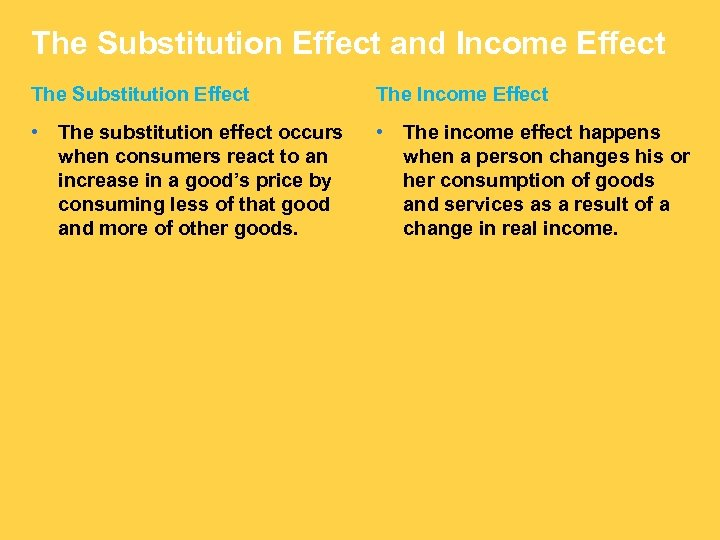The Substitution Effect and Income Effect The Substitution Effect The Income Effect • The substitution effect occurs when consumers react to an increase in a good’s price by consuming less of that good and more of other goods. • The income effect happens when a person changes his or her consumption of goods and services as a result of a change in real income.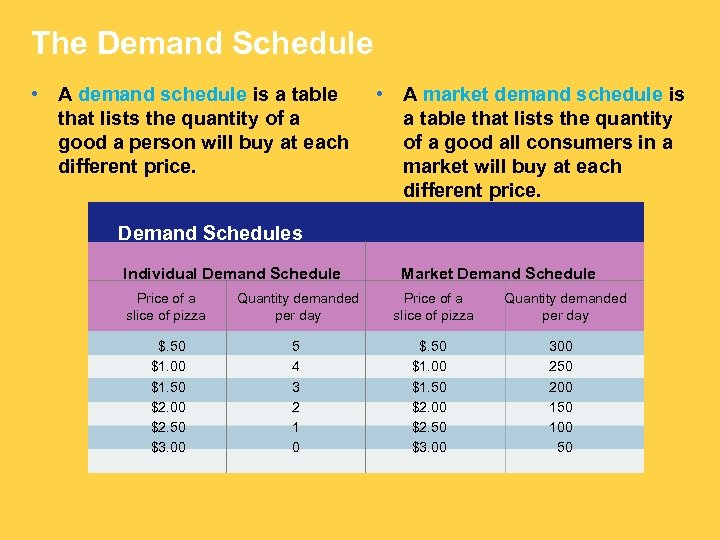The Demand Schedule • A demand schedule is a table that lists the quantity of a good a person will buy at each different price. • A market demand schedule is a table that lists the quantity of a good all consumers in a market will buy at each different price. Demand Schedules Individual Demand Schedule Price of a slice of pizza Quantity demanded per day \$. 50 \$1. 00 \$1. 50 \$2. 00 \$2. 50 \$3. 00 5 4 3 2 1 0 Market Demand Schedule Price of a slice of pizza \$. 50 \$1. 00 \$1. 50 \$2. 00 \$2. 50 \$3. 00 Quantity demanded per day 300 250 200 150 100 50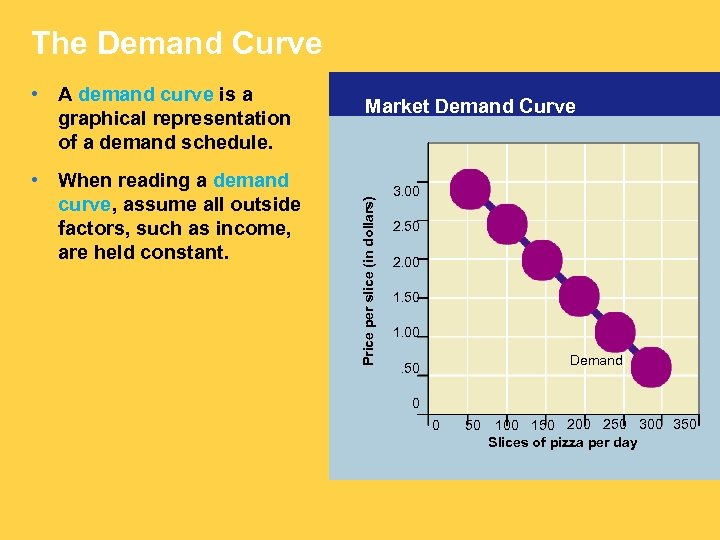The Demand Curve • When reading a demand curve, assume all outside factors, such as income, are held constant. Market Demand Curve Price per slice (in dollars) • A demand curve is a graphical representation of a demand schedule. 3. 00 2. 50 2. 00 1. 50 1. 00 Demand . 50 0 0 50 100 150 200 250 300 350 Slices of pizza per day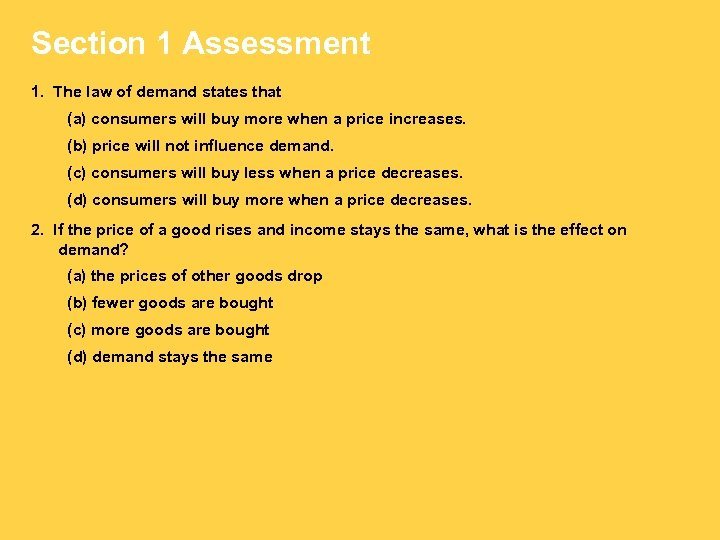Section 1 Assessment 1. The law of demand states that (a) consumers will buy more when a price increases. (b) price will not influence demand. (c) consumers will buy less when a price decreases. (d) consumers will buy more when a price decreases. 2. If the price of a good rises and income stays the same, what is the effect on demand? (a) the prices of other goods drop (b) fewer goods are bought (c) more goods are bought (d) demand stays the same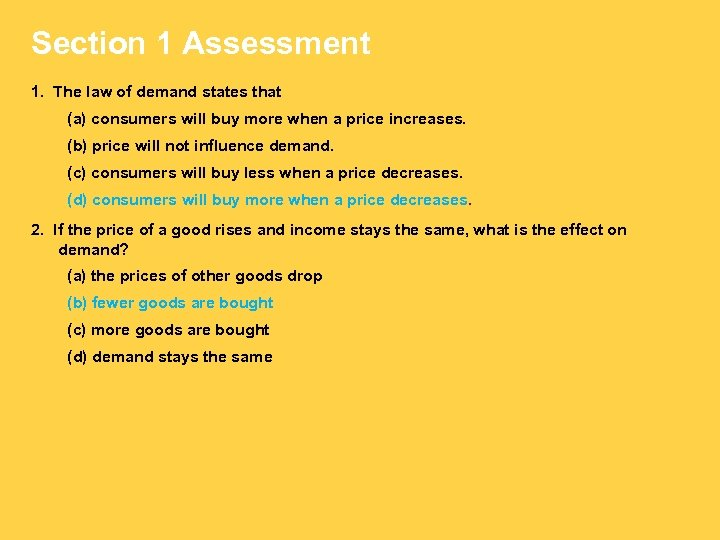Section 1 Assessment 1. The law of demand states that (a) consumers will buy more when a price increases. (b) price will not influence demand. (c) consumers will buy less when a price decreases. (d) consumers will buy more when a price decreases. 2. If the price of a good rises and income stays the same, what is the effect on demand? (a) the prices of other goods drop (b) fewer goods are bought (c) more goods are bought (d) demand stays the same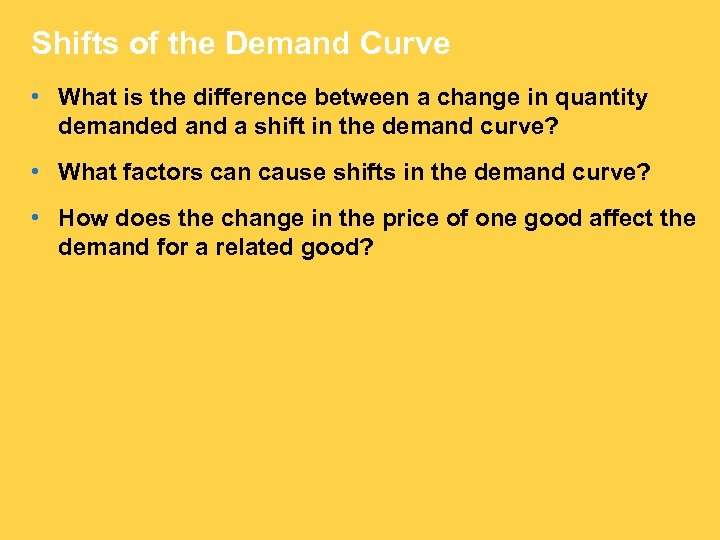Shifts of the Demand Curve • What is the difference between a change in quantity demanded and a shift in the demand curve? • What factors can cause shifts in the demand curve? • How does the change in the price of one good affect the demand for a related good?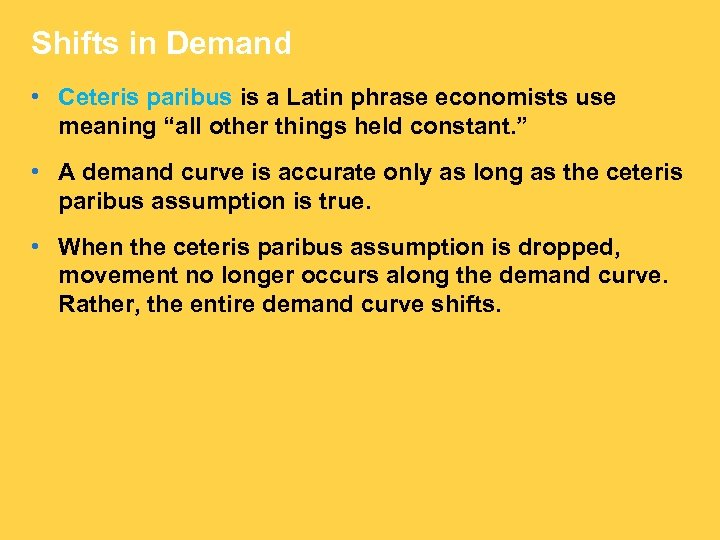Shifts in Demand • Ceteris paribus is a Latin phrase economists use meaning “all other things held constant. ” • A demand curve is accurate only as long as the ceteris paribus assumption is true. • When the ceteris paribus assumption is dropped, movement no longer occurs along the demand curve. Rather, the entire demand curve shifts.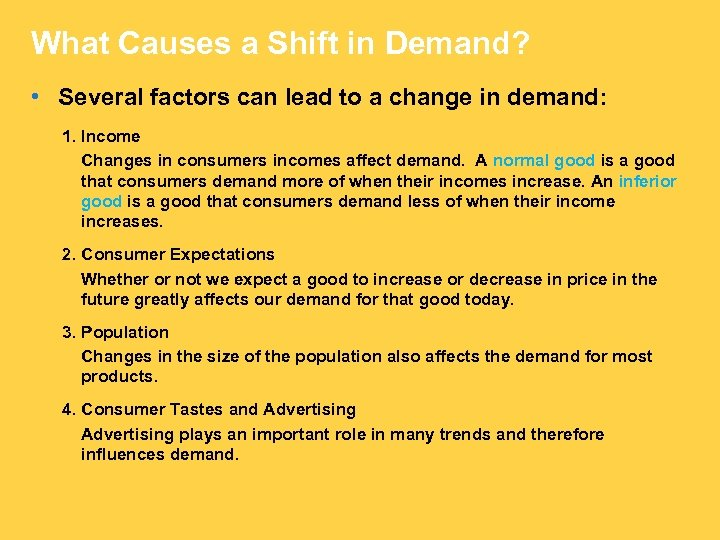What Causes a Shift in Demand? • Several factors can lead to a change in demand: 1. Income Changes in consumers incomes affect demand. A normal good is a good that consumers demand more of when their incomes increase. An inferior good is a good that consumers demand less of when their income increases. 2. Consumer Expectations Whether or not we expect a good to increase or decrease in price in the future greatly affects our demand for that good today. 3. Population Changes in the size of the population also affects the demand for most products. 4. Consumer Tastes and Advertising plays an important role in many trends and therefore influences demand.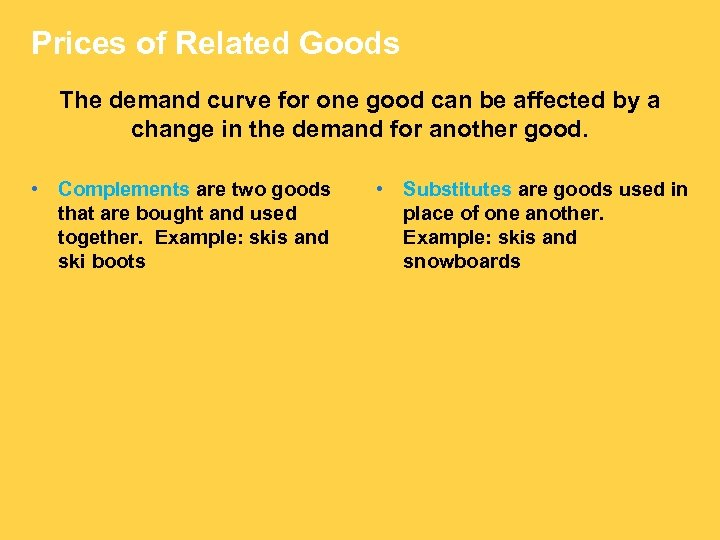Prices of Related Goods The demand curve for one good can be affected by a change in the demand for another good. • Complements are two goods that are bought and used together. Example: skis and ski boots • Substitutes are goods used in place of one another. Example: skis and snowboards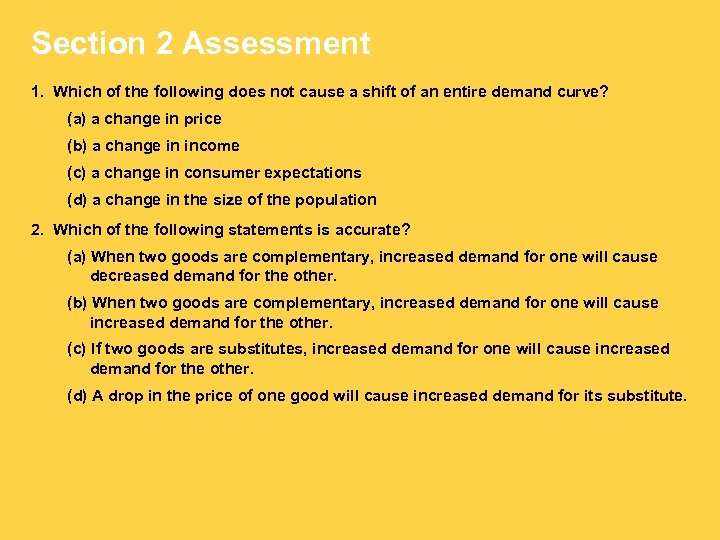Section 2 Assessment 1. Which of the following does not cause a shift of an entire demand curve? (a) a change in price (b) a change in income (c) a change in consumer expectations (d) a change in the size of the population 2. Which of the following statements is accurate? (a) When two goods are complementary, increased demand for one will cause decreased demand for the other. (b) When two goods are complementary, increased demand for one will cause increased demand for the other. (c) If two goods are substitutes, increased demand for one will cause increased demand for the other. (d) A drop in the price of one good will cause increased demand for its substitute.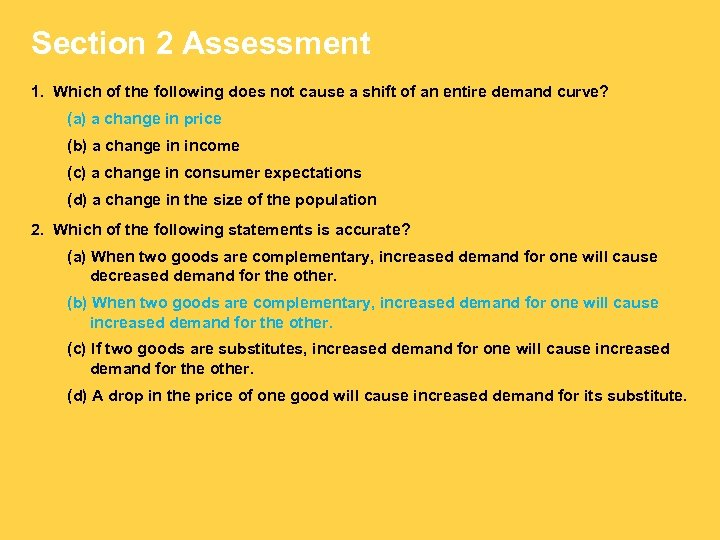Section 2 Assessment 1. Which of the following does not cause a shift of an entire demand curve? (a) a change in price (b) a change in income (c) a change in consumer expectations (d) a change in the size of the population 2. Which of the following statements is accurate? (a) When two goods are complementary, increased demand for one will cause decreased demand for the other. (b) When two goods are complementary, increased demand for one will cause increased demand for the other. (c) If two goods are substitutes, increased demand for one will cause increased demand for the other. (d) A drop in the price of one good will cause increased demand for its substitute.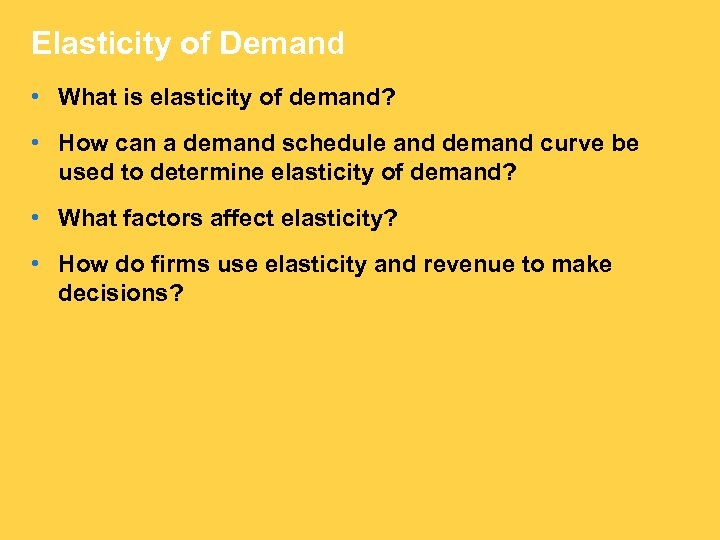Elasticity of Demand • What is elasticity of demand? • How can a demand schedule and demand curve be used to determine elasticity of demand? • What factors affect elasticity? • How do firms use elasticity and revenue to make decisions?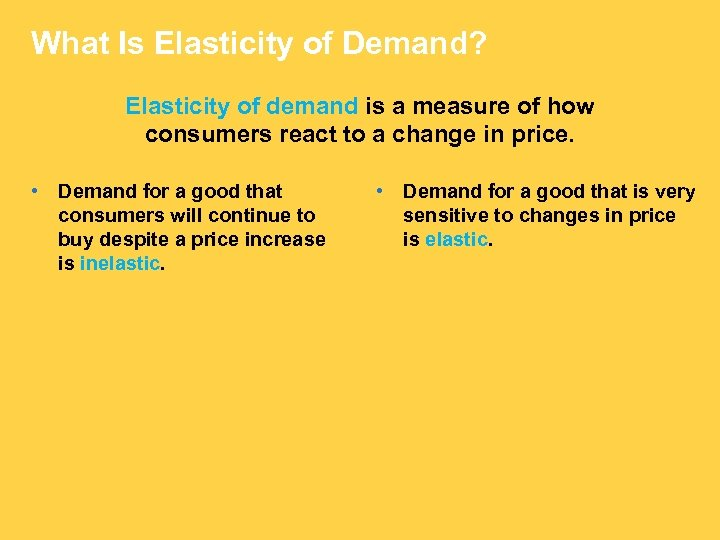What Is Elasticity of Demand? Elasticity of demand is a measure of how consumers react to a change in price. • Demand for a good that consumers will continue to buy despite a price increase is inelastic. • Demand for a good that is very sensitive to changes in price is elastic.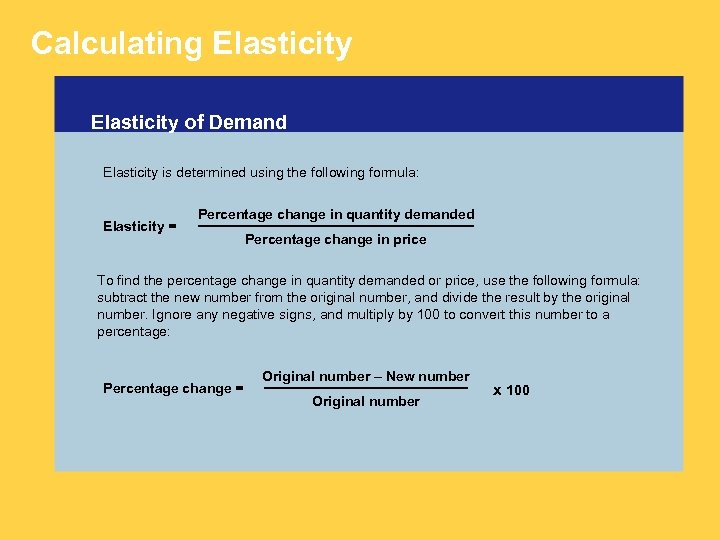Calculating Elasticity of Demand Elasticity is determined using the following formula: Elasticity = Percentage change in quantity demanded Percentage change in price To find the percentage change in quantity demanded or price, use the following formula: subtract the new number from the original number, and divide the result by the original number. Ignore any negative signs, and multiply by 100 to convert this number to a percentage: Percentage change = Original number – New number Original number x 100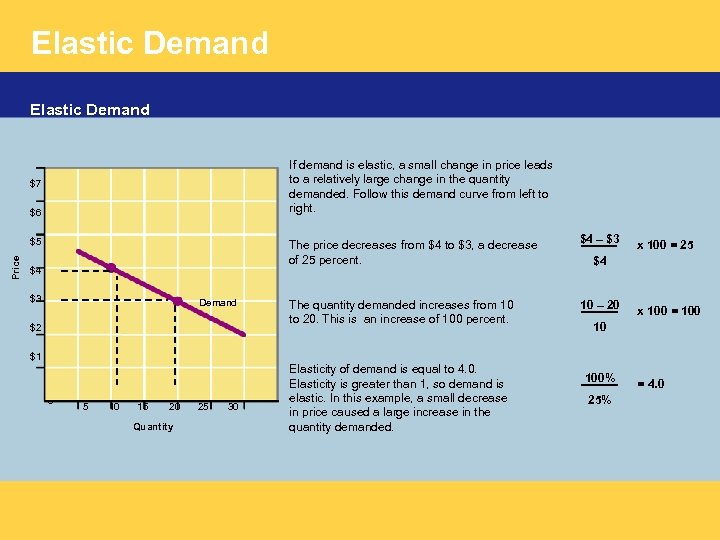Elastic Demand If demand is elastic, a small change in price leads to a relatively large change in the quantity demanded. Follow this demand curve from left to right. \$7 \$6 The price decreases from \$4 to \$3, a decrease of 25 percent. Price \$5 \$4 \$3 Demand \$2 \$4 – \$3 The quantity demanded increases from 10 to 20. This is an increase of 100 percent. 10 – 20 x 100 = 25 \$4 x 100 = 100 10 \$1 0 5 10 15 20 Quantity 25 30 Elasticity of demand is equal to 4. 0. Elasticity is greater than 1, so demand is elastic. In this example, a small decrease in price caused a large increase in the quantity demanded. 100% 25% = 4. 0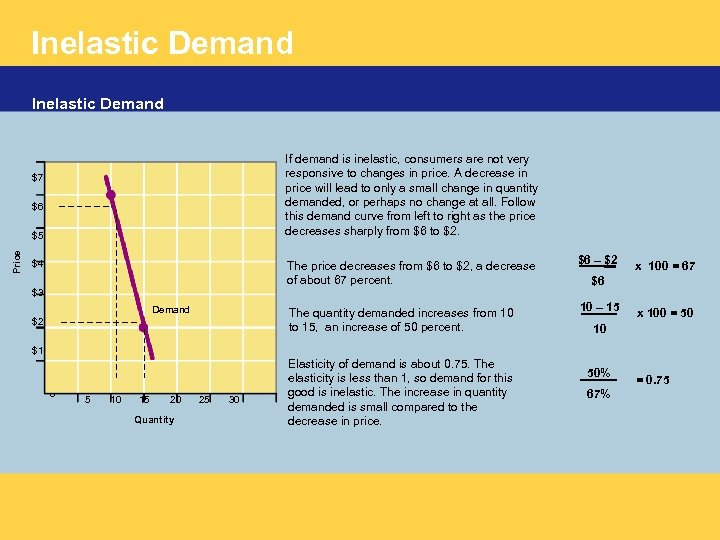Inelastic Demand If demand is inelastic, consumers are not very responsive to changes in price. A decrease in price will lead to only a small change in quantity demanded, or perhaps no change at all. Follow this demand curve from left to right as the price decreases sharply from \$6 to \$2. \$7 \$6 Price \$5 The price decreases from \$6 to \$2, a decrease of about 67 percent. \$6 – \$2 The quantity demanded increases from 10 to 15, an increase of 50 percent. \$4 10 – 15 \$3 Demand \$2 x 100 = 67 \$6 x 100 = 50 10 \$1 0 5 10 15 20 Quantity 25 30 Elasticity of demand is about 0. 75. The elasticity is less than 1, so demand for this good is inelastic. The increase in quantity demanded is small compared to the decrease in price. 50% 67% = 0. 75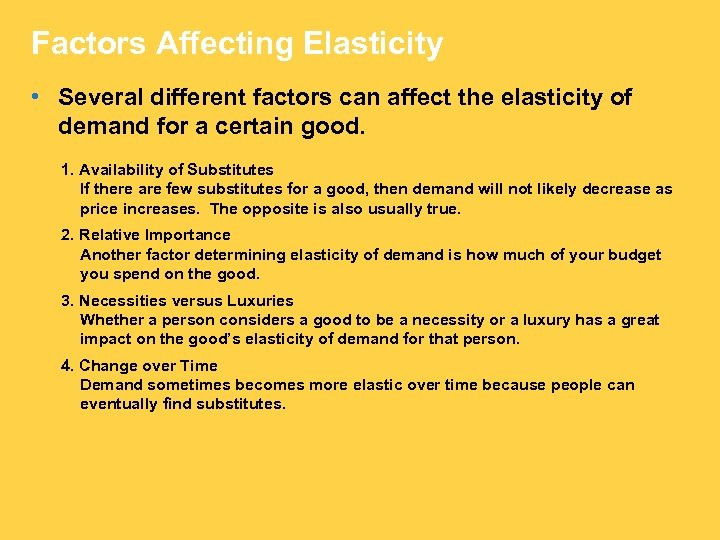Factors Affecting Elasticity • Several different factors can affect the elasticity of demand for a certain good. 1. Availability of Substitutes If there are few substitutes for a good, then demand will not likely decrease as price increases. The opposite is also usually true. 2. Relative Importance Another factor determining elasticity of demand is how much of your budget you spend on the good. 3. Necessities versus Luxuries Whether a person considers a good to be a necessity or a luxury has a great impact on the good’s elasticity of demand for that person. 4. Change over Time Demand sometimes becomes more elastic over time because people can eventually find substitutes.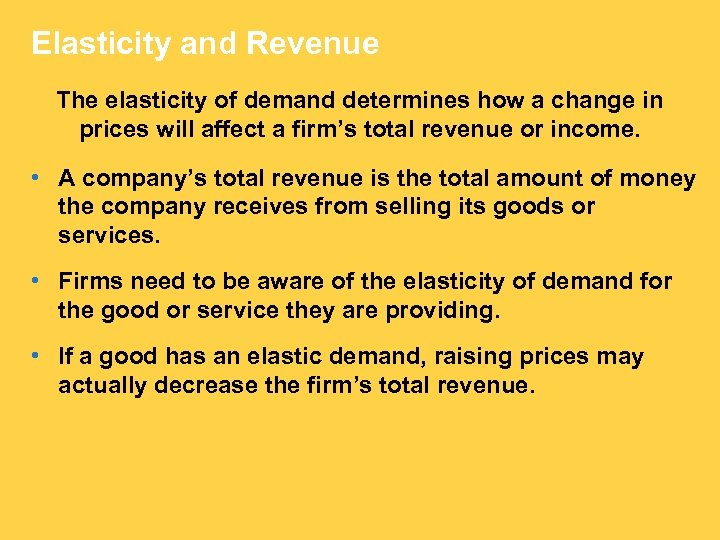Elasticity and Revenue The elasticity of demand determines how a change in prices will affect a firm’s total revenue or income. • A company’s total revenue is the total amount of money the company receives from selling its goods or services. • Firms need to be aware of the elasticity of demand for the good or service they are providing. • If a good has an elastic demand, raising prices may actually decrease the firm’s total revenue.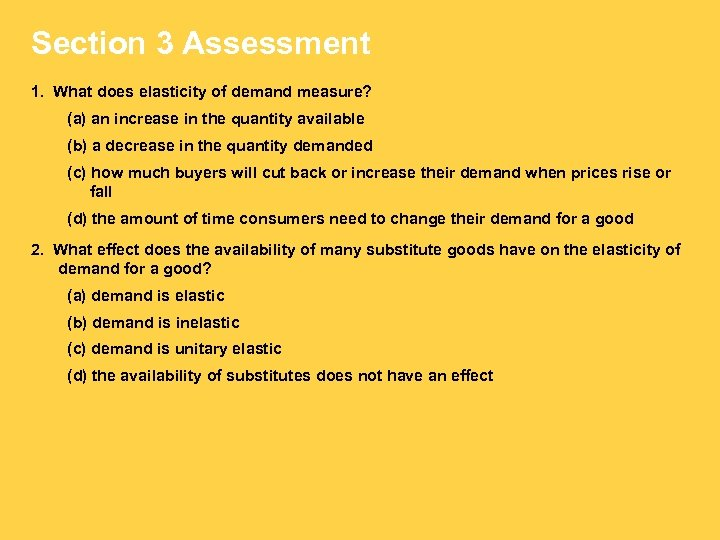Section 3 Assessment 1. What does elasticity of demand measure? (a) an increase in the quantity available (b) a decrease in the quantity demanded (c) how much buyers will cut back or increase their demand when prices rise or fall (d) the amount of time consumers need to change their demand for a good 2. What effect does the availability of many substitute goods have on the elasticity of demand for a good? (a) demand is elastic (b) demand is inelastic (c) demand is unitary elastic (d) the availability of substitutes does not have an effect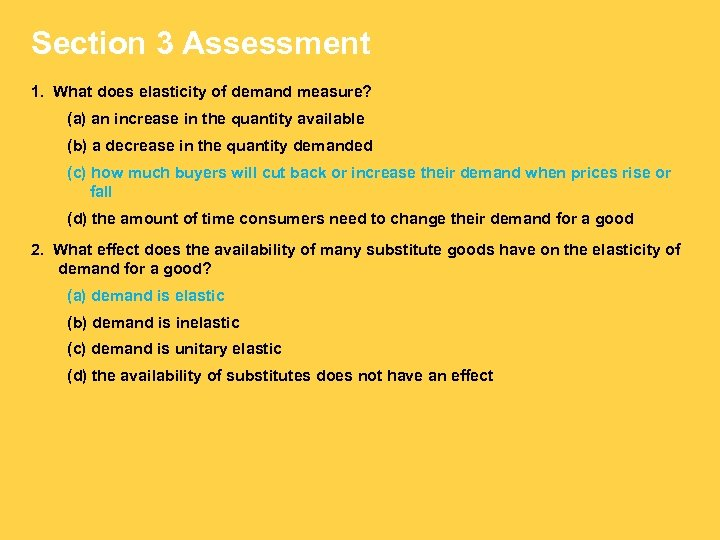Section 3 Assessment 1. What does elasticity of demand measure? (a) an increase in the quantity available (b) a decrease in the quantity demanded (c) how much buyers will cut back or increase their demand when prices rise or fall (d) the amount of time consumers need to change their demand for a good 2. What effect does the availability of many substitute goods have on the elasticity of demand for a good? (a) demand is elastic (b) demand is inelastic (c) demand is unitary elastic (d) the availability of substitutes does not have an effect